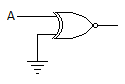# Electronics and Communication Engineering - Digital Electronics - Discussion

11.

For the gate in the given figure the output will be[A]. 0 [B]. 1 [C]. A [D]. A

Explanation:

If A = 0, Y = 1 and A = 1, Y = 0 Therefore Y = A.

 Vamsikrishna said: (Dec 1, 2014) Why can't we choose option 'a'?

 Raja said: (Jan 13, 2015) It's actual not gate so a=0; y=1, a=1; y=0.

 Reshmi said: (Jul 20, 2015) Here the gate is XNOR. Output will be one when both inputs are same i,e, 00=1 and 11=1. Here 2nd input is always AND so zero. So possibilities are A become 1 or 0. (if A=1, input 10=0 ie A' if A=0 input 00=1 since gate is xnor i.e A').

 Vicky said: (May 27, 2016) Formula XNOR = A(BAR) B(BAR) + AB. So, from the given question: A (bar). 1 + A (0) = A(BAR).

 Anusha. Byravaram said: (Aug 25, 2017) If we take both inputs as 0 or both as 1, then the answer will be change. Then why you have taken only 0 and 1, 1 and 0.?

 Ayush Kumar said: (Nov 4, 2017) Right answer is D because A^0^ + A.0 = A^.

 Dhammapriy said: (Mar 19, 2018) Actually, when you tie one of the inputs of XNOR gate to logic 0 then XNOR gate behaves as a inverter.

 Habiba said: (May 18, 2018) Please explain it clearly.

 M S Kamalanadhan said: (Sep 6, 2018) It is XNOR gate so when both ip's are same op is 1.

 V Naga Pranavi said: (Sep 20, 2018) It is the XNOR gate. One input is A. Here the other input(say B) is 0. since it is grounded. When A=0 B=0, O/p Y='1'. when A=1 B=0 O/p Y='0'; i.e; Y=A'.

 Vijaya said: (Dec 5, 2018) Well said. Thank you @Reshmi.

 Bhargav said: (Dec 8, 2020) Nice explanation, Thanks @V Naga Pranavi.